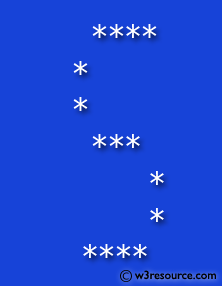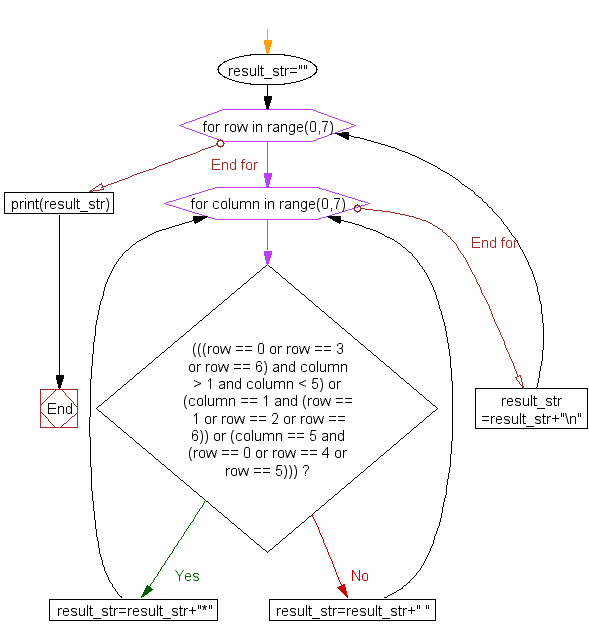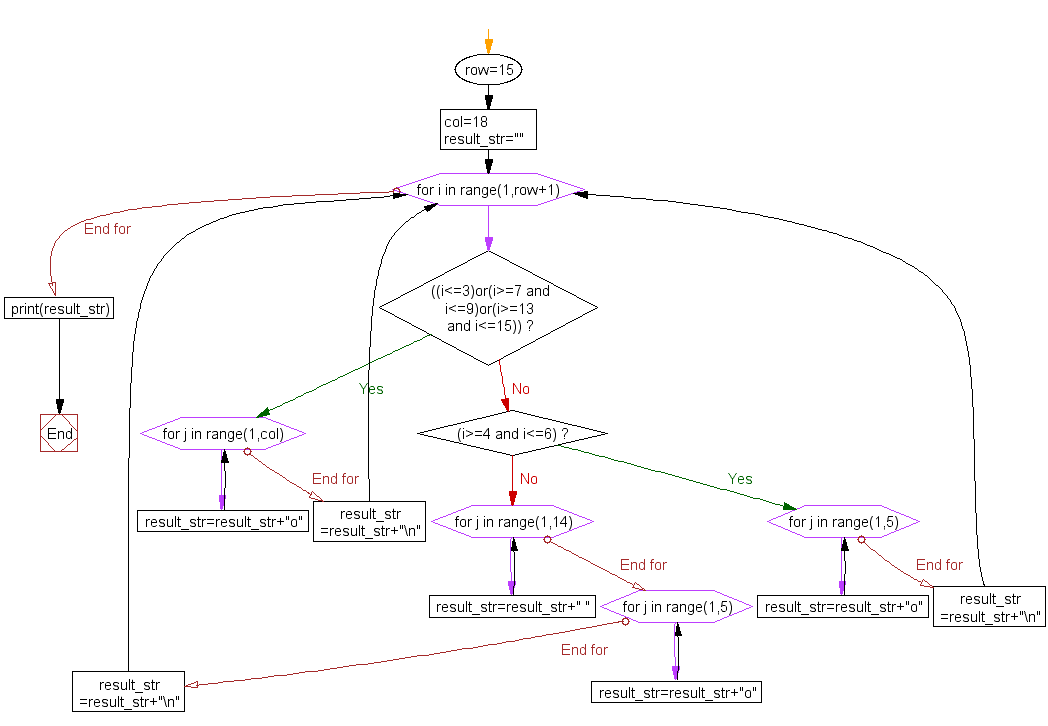﻿ Python Exercise: Print alphabet pattern S - w3resource

# Python Exercise: Print alphabet pattern S

## Python Conditional: Exercise - 26 with Solution

Write a Python program to print the following pattern 'S'.

Pictorial Presentation:Sample Solution:

Python Code:

``````# Initialize an empty string named 'result_str'
result_str = ""

# Loop through rows from 0 to 6 using the range function
for row in range(0, 7):
# Loop through columns from 0 to 6 using the range function
for column in range(0, 7):
# Check conditions to determine whether to place '*' or ' ' in the result string

# If conditions are met, place '*' in specific positions based on row and column values
if (((row == 0 or row == 3 or row == 6) and column > 1 and column < 5) or
(column == 1 and (row == 1 or row == 2 or row == 6)) or
(column == 5 and (row == 0 or row == 4 or row == 5))):
result_str = result_str + "*"  # Append '*' to the 'result_str'
else:
result_str = result_str + " "  # Append space (' ') to the 'result_str'

result_str = result_str + "\n"  # Add a newline character after each row in 'result_str'

# Print the final 'result_str' containing the pattern for the first shape
print(result_str)

# Define variables 'row' and 'col' with values 15 and 18 respectively
row = 15
col = 18
result_str = ""  # Reinitialize the 'result_str' to an empty string

# Loop through values from 1 to 'row' using the range function
for i in range(1, row+1):
# Check conditions to determine the pattern for each row

# If conditions are met, append 'o' to the 'result_str' in specific positions
if ((i <= 3) or (i >= 7 and i <= 9) or (i >= 13 and i <= 15)):
for j in range(1, col):
result_str = result_str + "o"  # Append 'o' to the 'result_str'
result_str = result_str + "\n"  # Add a newline character after each row in 'result_str'
elif (i >= 4 and i <= 6):
for j in range(1, 5):
result_str = result_str + "o"  # Append 'o' to the 'result_str'
result_str = result_str + "\n"  # Add a newline character after each row in 'result_str'
else:
for j in range(1, 14):
result_str = result_str + " "  # Append space (' ') to the 'result_str'
for j in range(1, 5):
result_str = result_str + "o"  # Append 'o' to the 'result_str'
result_str = result_str + "\n"  # Add a newline character after each row in 'result_str'

# Print the final 'result_str' containing the pattern for the second shape
print(result_str)
```
```

Sample Output:

```
****
*
*
***
*
*
****

ooooooooooooooooo
ooooooooooooooooo
ooooooooooooooooo
oooo
oooo
oooo
ooooooooooooooooo
ooooooooooooooooo
ooooooooooooooooo
oooo
oooo
oooo
ooooooooooooooooo
ooooooooooooooooo
ooooooooooooooooo
```

Flowchart Part-1:Flowchart Part-2:Python Code Editor:

Have another way to solve this solution? Contribute your code (and comments) through Disqus.

What is the difficulty level of this exercise?

Test your Programming skills with w3resource's quiz.

﻿